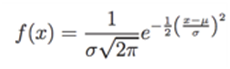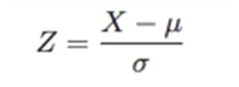# Probability Distributions Assignment Help

In the field of Inferential Statistics, for the values of the parameters which are basically descriptions of the summary of values, assumptions are made using sample data. The parameters were there fly unknown. However, her these descriptions of summaries called parameters are known. While the point of inferential statistics becomes redundant considering parameters are never know but use this types to technique to compare the observation to what we expect in the future.

## Probability distributions

To understand the term probability, we first consider an example, a hypothetical experiment, in which certain events are occurred repeatedly. Here, observations are made and what is noted is that the outcomes may or may not be same for every observation. No we can say that probability is defined by its relativity to frequency in the long run.

We can define it formally as: “The probability of a particular outcome is the proportion of times that outcome would occur in a long run of repeated observations.”

We can consider a long sequence of flipping of coins which can have two possibilities: tails facing upwards and head facing downwards, or heads facing upwards and tails facing downwards. However, if there’s is balance in the outcomes, then according to law of large numbers, that even though its imbalanced, the more number of flips of sequences will send the possibility of getting heads or tails towards half (1/2). Hence, probability of tails or heads is 1/2.

In a lot of the scenarios, the outcomes of the variables we are observing are numerate and in the long run, with repeated observations, can take two or more values. These variables are called random variables.

Formally, we can define a random variable as “A random variable is a variable whose value is a numerical outcome of a random phenomenon.”

These can be denoted by capital letters, generally towards the latter end of the alphabet. For example X or Y. Since some outcomes may be more likely to occur, all the possible outcomes and their probability are listed in the probability distribution.

A probability is attached to each value of the discrete random variable X that is possible in the probability distribution. The sum of all probabilities show always equal 1 and individual probabilities can only lie between 0 and 1. For example: For a random variable X, we denote outcomes of these as : xi , i = 1, 2, . . . , k. and probability of which will be P(X = xi) = P(xi) = pi. So,considering the total probability is always 1 and each individual probability lies between 0 and 1.

This discrete random variable when represented in the probability distribution cane be formally defined as “A discrete random variable X has a countable number of possible values. The probability distribution of X lists the values and their probabilities:Here, to depict it graphically, we use a histogram to show probability distribution of the discrete random variable and then carry on to finding the probability of each observation ( P(X ≤ xi) or P(xi ≤ X ≤ xj ), i ≤ j] ) by summing the probabilities of them.

We can show this in an example: A professor of a big group pf students grades 25% of everyone a 5 which is excellent, 15% of everyone a 4 which stands for very good, 30% of everyone a 3 which means good, 20% of everyone a 2 who depicts satisfactory results and 10% of all a 1 which refers to their work being sufficient. If we randomly choose a student’s grade and assign it an X . It can be either 5,4,3,2 or 1. through the above formula we can find out the probability distribution of X along with contrasting a histogram.

Moving on, when we consider continuous random variables, for example x, all the values between a and b which are intervals are considered. Here we use x1, x2 which the condition that x1 is less than x2 depict the outcomes which are possible for continuous random variables with values between the intervals a and b.

We can summarise that :concluding that both x1 and x2 belong to the interval a and b . Then x1 and x2 becoming intervals themselves are assigned a probability each by the probability distribution. Like we said before the possibility that X failed between x1 and x2 lies between 0 and 1.

Resulting with the following equation:

0 ≤ P(x1 ≤ X ≤ x2) ≤ 1 and P(a ≤ X ≤ b) = 1.

Formally we can define the continuous random variable when represent in a probability distribution as: “A continuous random variable X takes all values in an interval of numbers [a, b]. The probability distribution of X describes the probabilities P(x1 ≤ X ≤ x2) of all possible intervals of numbers [x1, x2].

The probabilities P(x1 ≤ X ≤ x2) must satisfy two requirements:

1. For every interval [x1, x2], the probability P(x1 ≤ X ≤ x2) is a number between 0 and 1.

2. P(a ≤ X ≤ b) = 1.

Here, in this case of continuous random variable, probabilities are assigned to intervals and not to outcomes of individual observations. Actually , a probability of 0 is attached o every individual outcome in continuous probability distributions.

To depict it graphically, A density curve is used with is smooth continuous curve with 1 being the area under it which represents the distribution.

We can look at this in the example mentioned below:

Example:This is the figure depicting the probability distribution. In case of continuous random variables, probabilities are assigned to the areas under the density curve.

### Normal Distribution

When this density curve which is the graphical representation of a continuous random variable is used, it has a normal distribution. It is one of the most important types of distributions in the entire topic of statistics. It is also due to its simplicity since it is known to approximate well with various another variable distributions. In cases that we often refer to as the variable to be approximately normally distributed when histograms derived out of the sample data are often bell-shapes same as the density curve. However, it is also when the data is not bell shapes that other methods in inferential statistics use properties of normal distribution.

The two parameters that are assigned to a continuous random variable are: the mean µ and the standard deviation σ.

It can be formally defined as “ A continuous random variable X is said to be normally distributed or to have a normal distribution if its density curve is a symmetric, bell-shaped curve, characterized by its mean µ and standard deviation σ. For each fixed number z, the probability concentrated within interval [µ − zσ, µ + zσ] is the same for all normal distributions. Particularly, the probabilities hold. A random variable X following normal distribution with a mean of µ and a standard deviation of σ is denoted by X  N(µ, σ). :

P(µ − σ < X < µ + σ) = 0.683 P(µ − 2σ < X < µ + 2σ) = 0.954

P(µ − 3σ < X < µ + 3σ) = 0.997”

Moreover to calculate the area we need to determine the heft of the density curve. This can be given by the density functionWe also need to note that that  µ and σ completely determine the density function.

An example of The normal distribution is given below:Formally the Standard normal table can be defined as “ A continuous random variable Z is said to have a standard normal distribution if Z is normally distributed with mean µ = 0 and standard deviation σ = 1, i.e., Z  N(0, 1)”

To estimate this random variable Z, a standard normal table is used. To value of z is given to the area to the left under the density curve by the standard normal table. This can be denoted as :

P(Z ≤ z) = Area under curve to the left of z.

And for the probability for an interval (a,b):

P(a ≤ Z ≤ b) = [Area to left of b] − [Area to left of a]

There are various properties from the symmetry of the standard normal table that can be observed.

given by P(Z ≤ 0) = 0.5, P(Z ≤ −z) = 1 − P(Z ≤ z) = P(Z ≥ z)

We can denote this in the example below:

Example: to calculate the probability of P(-0.255 < Z < 2.60) and to see if P (Z > z) = 0.35 is satisfied by a value z and to find it.

We can solve this by following the below methods:

If X ∼ N(µ, σ) is what X is distributed as, then to find the variable which is standardised we use :which has the standard normal distribution and when X ∼ N(µ, σ), is what X is distributed as then:standard normal distribution represented by Z.